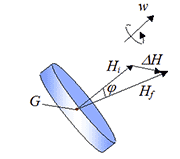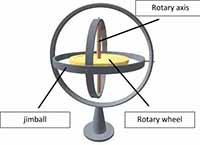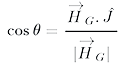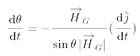# Gyroscope stability

According to the equations and studies done at the angular momentum, the angular momentum vector variations of the rigid body in the time interval $t_i$ to $t_f$ are calculated as follows. The parameter $H_f$ represents the angular momentum vector at the final moment of $t_f$. The angular momentum vector at the initial moment of ti is also $H_i$. The vector M also indicates the external moments entering the rigid body.

Although the above relation is defined for a rigid body, it can also be used for any system of particles. To prove this you can refer to classical books in the field of mechanical engineering. This relationship is used in two cases. One when the origin of the xyz axes is located at the center of the mass G of the rigid body. In the latter case, this source can be placed at the O point that is fixed on the rigid body (if any). This section is used from the first mode. Therefore, torques, inertial values and angular momentum will be measured relative to the G point. Here, to show the gyroscope stability, consider the peg body as a disk that has a symmetry axis. This object is in the given moment at an angular velocity ω.In the above figure, the angular momentum vector variation between ti and tf times is shown with ΔH. According to the previous relation, this value is equal to the external impact Here, and with respect to the equations with constant ΔH, the decrease in the angle φ increases the amount of Hi. That is, the greater the magnitude of the initial angular momentum, the angle φ will be smaller to change the ΔH to a constant value. Consequently, the vector size of the angular momentum H is proportional to the angular velocity vector ω. In other words, the faster the object rotates, the angle φ becomes smaller. If no external torque is entered into the object, $H_i=H_f$ and the angle φ will be zero. In this case, the motion of the object is without torque. Therefore, the size and direction of the angular momentum vector are fixed and the angular momentum is constant. You imagine that in an asymmetric body that has a non-torque motion motion, the axis of the precession motion is matched to the angular momentum vector. The precession axis determines the orientation of the object. As a result, any small change in the vector direction of the angular momentum causes a slight change in the direction of the object. After the external impact is applied, the motion of the body again becomes torque. In this research, when a body that has axial symmetry and a high speed (without torque) spins around itself, an external impact is invoked, the body can maintain the axial movement of the present with a slight change.

# Application of Gyroscopic Effects in the Real World

Considering the nature of the gyroscope’s operation, it shows why, for guidance, a rotating disk (powered by a motor) is used within a metal frame (Jimball). The rotating disk is mounted inside the metal frame so that no external torque can be entered. Therefore, its orientation, except for a small amount, will not change. This causes the gyroscope to be used abundantly in navigation systems for ships and boats. In this case, the direction of the gyroscope remains unchanged, even if the direction of the ship moves. The following figure shows a gyro-jimball system sample.Here, with a simple look, the gyroscope’s stability can be checked in the projectile’s motion. As a familiar example, consider the ball used in American football, which has a central symmetry, and circles itself after the launch. Suppose this ball is thrown right. In this case, the direction of its longitudinal axis does not change during the flight, as shown below. The rotation of the ball in its distance in response to aerodynamic forces creates a gyroscopic effect. In this example, with a combination of gyroscopic acceleration, we have aerodynamic forces due to Drag and Lift (Force). Here we are not looking at the analysis of this complex problem, and this example is presented as just an application of gyroscope’s real-world effect.In the following, we will examine another issue. Consider the body piece that has no torque. In this case, from the observer’s point of view in the bare coordinate system, it seems that the axis of motion is matched to the angular momentum vector. While we know that the axis is constant, its size and direction do not change.

# Pure spin mathematical analysis in gyroscope

Once again, in this mathematical calculus. Consider the following figure that defines the axes of the local xyz coordinate.We want to find a relationship between the angle $\theta$ and the vectors $H_G$ and $\omega_s$. Using the internal multiplication, the following equation is easily obtained.In this equation, $H_G$ is the angular momentum around center G. With a time derivation of this relation in time, the following result is obtained.Here, by inserting the expression  dj//dt= (omegaxxj) / (2a)  the above relation and using the concept of external multiplication, the previous relation can be written as follows.

According to the obtained equation, when the values of $I_x$ and $I_z$ are equal (both equal to $I_w$ ), dθ / dt will be zero. Like what happens to a spinning object in space. Now assume that the angle α is zero. In this case, the propulsion axis is placed on the HG angular momentum vector. As a result, and θ will be constant

In this study, we want to calculate $\omega_s$ and $\omega_p$ by using the above results. Since the angle θ is always constant, the angular momentum can be expressed as follows in the xyz coordinate system.

On the other hand, we have the following relationships beforehand.

$\large {\overrightarrow{H}}_G=I_x\omega_x\hat{i}+I_y\omega_y\hat{j}+I_z\omega_z\hat{k}\\~\\ \large {\overrightarrow{H}}_G=I_w\omega_x\hat{i}+I_{Gy}\omega_y\hat{j}+I_w\omega_z\hat{k}$\

Now, by comparing relations 5 and 6 with the help of geometric relations, the following equations are obtained.

Now solving these three equations we can find $\omega_s$ and $\omega_p$ by each other.

# Supplementary information on gyroscope

From the beginning of the discussion, and by check in of the equations, we have found that if the body is rotational at the angular momentum $\omega_s$ around its axis of symmetry, the axis of rotation and the vector of its angular momentum will be in the same direction. Now, this object may temporarily be exposed to an external torque. In this situation, the forward movement is added to the spin spin. From here on, the alignment axis aligns with the new vector of angular momentum. Euler motion equations must be used to calculate the new motion of the object. We also conclude that in a momentary motion, only the external force enters the body, the force that enters the center of mass. When it comes to motion without torque, it’s because there is no momentum that can rotate the body around its center of mass (G). As a result, the angular momentum around the center of mass will not change. For a better understanding of the subject, one can imagine that the center of rotation of the object lies in the center of mass. As you can see, according to simple mathematical relationships, the nature of the gyroscope is a complex subject, requiring deep attention. Hopefully, by studying this article, you have gained a good understanding of the performance of this physical effect, and this has given you an incentive to learn more about gyroscope concepts.